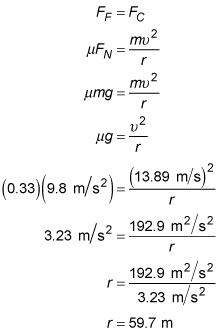##### Physics I: 501 Practice Problems For Dummies (+ Free Online Practice)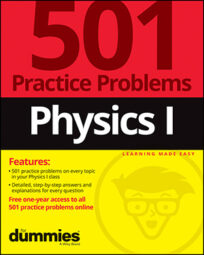Using physics, you can see how friction affects objects traveling on flat surfaces. For example, you can use the coefficient of friction to calculate the minimum allowable size of the curve in a road before a car starts to skid at a certain speed.

Here are some practice questions that you can try.

## Practice questions

1. What is the maximum speed that a 1,500-kilogram car can maintain without slipping when driving along a section of frictionless road with a radius of curvature of 25 meters?

2. Driving around a level bend, you notice that your car starts to skid just as you hit a speed of 50 kilometers per hour. If the coefficient of friction between the road and your car's tires is 0.33, what is the curve's radius of curvature — in meters — rounded to three significant digits?

The following are the answers to the practice questions:

1. 0 m/s

Without friction, a car can't maintain a curved path because no force is pointing toward the "center of the circle." Therefore, nothing provides the necessary centripetal force to keep the car going in a circular path.

2. 59.7 m

First, convert the given velocity to the desired units of meters per second: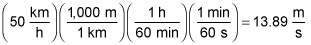Because the road is level (that is, completely horizontal), the only force that can possibly account for the centripetal force (FC) necessary to keep the car moving in a circular path is friction (FF). Therefore: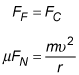The car is not accelerating in the vertical direction, so the net force in the vertical direction equals 0 newtons. Summing up the two forces in that direction, the normal force (FN) and the gravitational force (FG), results in: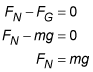Substituting this result into the formula relating the centripetal force to the frictional force yields: